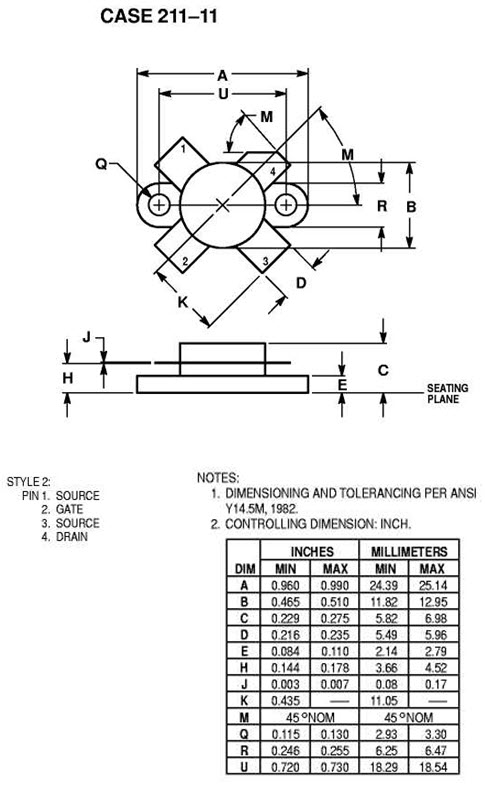# MRF150 - Silicon MOS N-Channel RF Power Transistor 150 W, up to 30 MHz, Enhancement ModeThe silicon MOS transistor is designed for professional transmitter applications in the HF frequency range.

Features:

• Guaranteed Performance at 30 MHz, 50 V:
• Power Gain: 17 dB Min
• Output Power: 150 W
• Efficiency: 45 % typ
Send request
Send request

## Absolute Maximum Ratings

Drain-Source Voltage VDSS 125 VDC
Gate-Source Voltage VGS ±40 VDC
Storage Temperature Range TSTG -65 tu +150 ºC
Thermal Resistance, Junction to Case RqJC 0.6 °C/W
Total Power Dissipation @Tc=25°C PD 300 W

## Parameters

ParameterSymbolMin.Typ.Max.Unit
Drain-Source Breakdown Voltage (IDS=100.0 mA, VGS=0 V) V(BR)DSS 125 VDC
Gate-Source Leakage Current (VGS=20 V, VDS=0 V) IGSS 1.0 uADC
Zero Gate Voltage Drain Leakage Current (VDS = 50 V, VGS=0 V) IDSS 5.0 mADC
Gate Threshold Voltage (VDS = 10 V, ID = 100 mA) VGS(TH) 1.0 5.0 VDC
Forward Transconductance (VDS = 10 V, ID = 5.0 A) GFS 4.0 7.0 mhos
Input Capacitance (VDS = 50 V, VGS=0 V, f = 1 MHz) CISS 400 pF
Output Capacitance (VDS = 50 V, VGS=0 V, f = 1 MHz) COSS 240 pF
Reverse Transfer Capacitance (VDS = 50 V, VGS=0 V, f = 1 MHz) CRSS 40 pF
Power Gain (f = 30 MHz VDD = 50 V, POUT = 150 W(PEP), lDQ = 250 mA) Gp 17 dB
Drain Efficiency (f = 30; 30.001 MHz, VDD = 50 V, POUT = 150 W(PEP), lDQ = 250 mA) hD   45 %
Intermodulation Distortion (VDD = 50 V, Pout = 150 W (PEP), f1 = 30 MHz, f2 = 30.001 MHz, IDQ = 250 mA)   -32 dB